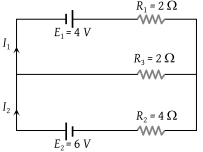# NEET Physics Current Electricity Questions Solved

In the circuit shown below E1 = 4.0 V, R1= 2 Ω, E2 = 6.0 V, R2 = 4 Ω and R3 = 2 Ω. The current I1 is(1) 1.6 A

(2) 1.8 A

(3) 1.25 A

(4) 1.0 A